# Use t`reeviewer` to Examine `HWTree` and `PriceTree` When Pricing European Callable Bond

This example demonstrates how to use `treeviewer` to examine tree information for a Hull-White tree when you price a European callable bond.

### Specify Input Parameters

Define the interest-rate curve information.

```Rates = [0.035; 0.042147; 0.047345; 0.052707]; ValuationDate = 'Jan-1-2019'; EndDates = {'Jan-1-2020'; 'Jan-1-2021'; 'Jan-1-2022'; 'Jan-1-2023'}; Compounding = 1;```

Define the callable bond instruments. The first instrument is the first entry in the arrays. So, for example, the first instrument has a strike price of \$98 and a maturity of January 1, 2022.

```Settle = '01-Jan-2019'; Maturity = {'01-Jan-2022'; '01-Jan-2023'}; CouponRate = {{'01-Jan-2021' .0425; '01-Jan-2023' .0450}}; OptType = 'call'; Strike = [98; 95]; ExerciseDates= {'01-Jan-2021'; '01-Jan-2022'}; Basis = 1;```

Define the volatility information and `HW` one-factor parameters.

```VolDates = ['1-Jan-2020'; '1-Jan-2021'; '1-Jan-2022'; '1-Jan-2023']; VolCurve = 0.05; AlphaDates = '01-01-2023'; AlphaCurve = 0.05; ```

### Build Hull-White One-Factor Tree

Use `hwtree` to build a one-factor tree.

```RateSpec = intenvset('ValuationDate', ValuationDate, 'StartDates', ValuationDate,... 'EndDates', EndDates,'Rates', Rates, 'Compounding', Compounding, 'Basis', Basis); HWVolSpec = hwvolspec(RateSpec.ValuationDate, VolDates, VolCurve, AlphaDates, AlphaCurve); HWTimeSpec = hwtimespec(RateSpec.ValuationDate, VolDates, Compounding); HWTimeSpec.Basis = Basis; HWT = hwtree(HWVolSpec, RateSpec, HWTimeSpec);```

### Price Both Callable Instruments

Use `optembndbyhw` to price the callable bond with embedded options.

```[Price, PriceTree] = optembndbyhw(HWT, CouponRate, Settle, Maturity, OptType, Strike,... ExerciseDates, 'Period', 1, 'Basis', Basis)```
```Price = 2×1 96.4131 92.9341 ```
```PriceTree = struct with fields: FinObj: 'HWPriceTree' tObs: [0 1 2 3 4] PTree: {[2x1 double] [2x3 double] [2x5 double] [2x7 double] [2x7 double]} ProbTree: { [0.1667 0.6667 0.1667] [0.0238 0.2218 0.5087 0.2218 0.0238] [0.0029 0.0473 0.2374 0.4247 0.2374 0.0473 0.0029] [0.0029 0.0473 0.2374 0.4247 0.2374 0.0473 0.0029]} ExTree: {[2x1 double] [2x3 double] [2x5 double] [2x7 double] [2x7 double]} ExProbTree: {[2x1 double] [2x3 double] [2x5 double] [2x7 double] [2x7 double]} ExProbsByTreeLevel: [2x5 double] Connect: { [2 3 4] [2 3 4 5 6]} ```

### Examine Hull-White Tree Structure

Use `treeviewer` to examine the Hull-White interest-rate tree that is the input for the embedded option pricer.

`treeviewer(HWT)`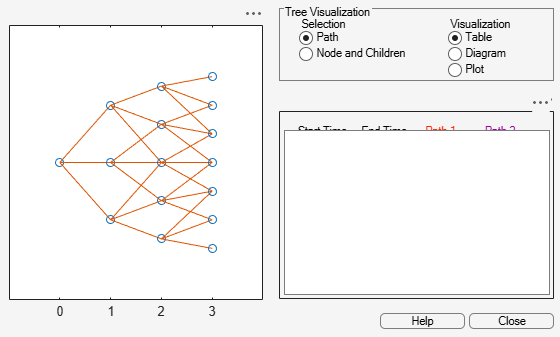The Hull-White tree has 4 levels of nodes. The root node is at t = `0`, three nodes are at t = `1`, five nodes are at t = `2`, and seven nodes are at t = `3`. Each node represents a particular state. In this case, the state is defined by the forward interest-rate curve, `HWT.FwdTree`. The combination of `HWT.FwdTree` and `HWT.Connec`t defines the tree structure. `FwdTree` contains the values of the forward interest rate at each node. The other fields contain other information relevant to interpreting the values in `FwdTree`. The most important are `VolSpec`, `TimeSpec`, and `RateSpec,` which contain the volatility, time structure, and rate structure information, respectively.

For example, `HWT.FwdTree` is:

`HWT.FwdTree`
```ans=1×4 cell array {[1.0350]} {[1.1457 1.0507 0.9635]} {[1.2639 1.1590 1.0629 0.9747 0.8938]} {[1.4003 1.2841 1.1776 1.0799 0.9903 0.9081 0.8328]} ```

If you display the nodes graphically with the forward rates superimposed it looks like: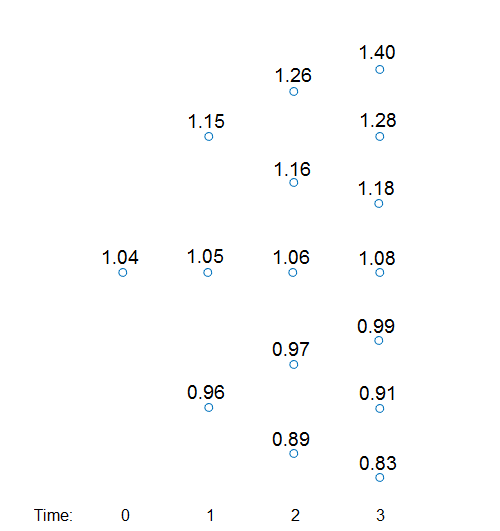You can use the `treeviewer` function to visualize the rates in the tree with `treeviewer(HWT)`. This function displays the structure of a Hull-White tree (`HWT`) in the left pane. The tree visualization in the right pane is blank. Visualize the actual interest-rate tree:

1. In the Tree Visualization pane, click Path and Diagram.

2. Select the first path by clicking the first node of the up branch at t `=` `1`.

3. Continue by clicking the up branch at the next node at t `=` `2`.

The following figures show the `treeviewer` path diagrams for these selections.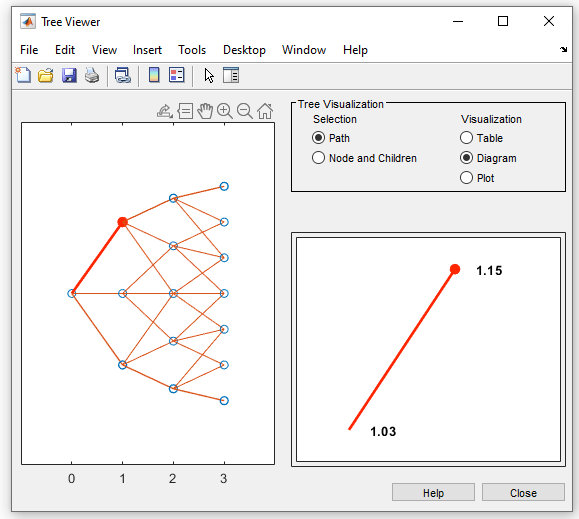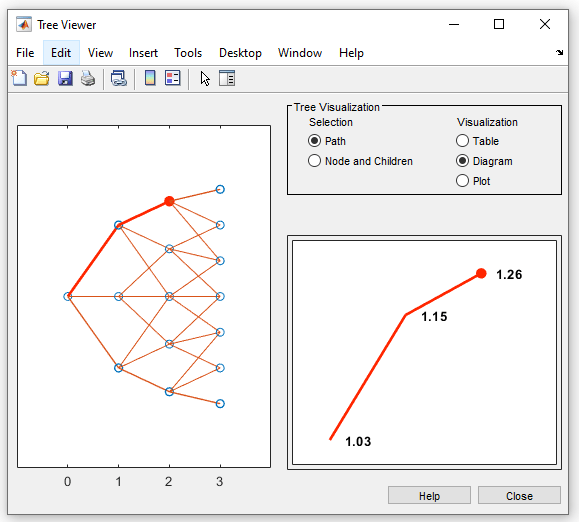4. Continue clicking all nodes in succession until you reach the end of the branch. The entire path you selected is highlighted in red.

5. Select a second path by clicking the first node of the lower branch at t `=` `1`. Continue clicking lower nodes as you did on the first branch. The second branch is highlighted in purple.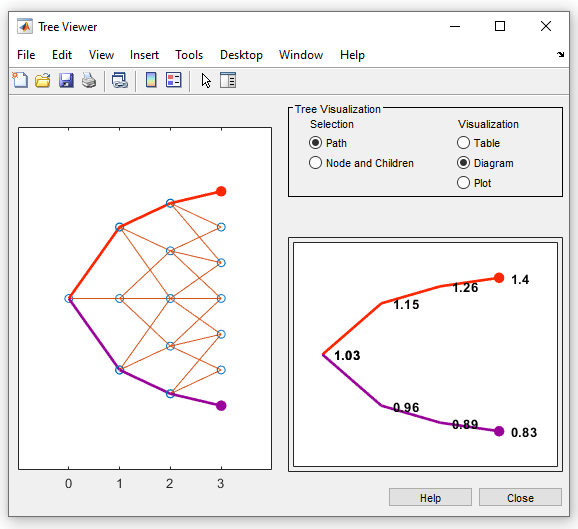Hull-White trees have two additional properties called `Probs` and `Connect`. The `Probs` property represents the transition probabilities and the `Connect` property defines how the nodes are connected together.

`Connect` Field

`HWT.Connect` describes the connectivity of the nodes of a given tree level to the nodes at the next tree level.

`HWT.Connect`
```ans=1×3 cell array {} {[2 3 4]} {[2 3 4 5 6]} ```

The first value of `HWT.Connect` corresponds to t = `0` for the root node and indicates that the root node connects to node 2 of the next level at t = `1`. To visualize this, consider the following connection illustration of the tree, where the node numbers have been superimposed above each node.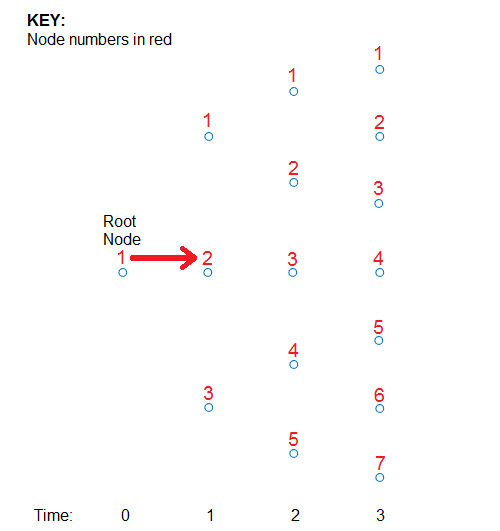Specifically, `HWT.Connect` represents the index of the node in the next tree level (t + `1`) that the middle branch of the node connects to.

The next entry in `HWT.Connect` at t = `1` is [`2,3,4`]. This means that node 1 at t = `1` has a middle branch with node 2 at t = `2`, node 2 at t = `1` has a middle branch with node 3 at t = `2`, and node 3 at t = `1` has a middle branch with node 4 at t = `2`. A graphic representation follows.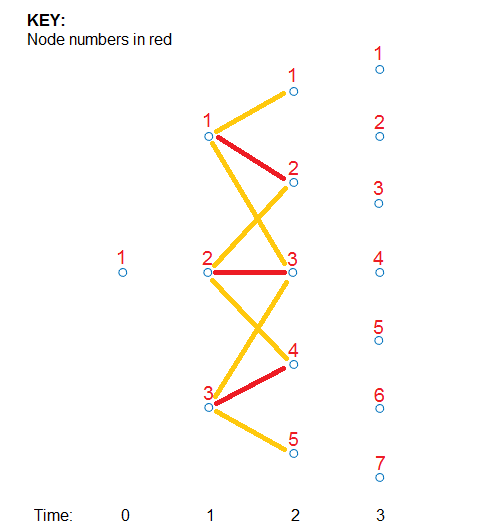The middle branch path, as explicitly defined in `HWT.Connect`, is colored red and the implicit upper and lower branch paths are colored yellow. Overlaying all paths defined in `HWT.Connect` as red and the implicit upper and lower branches as yellow produces the following tree structure.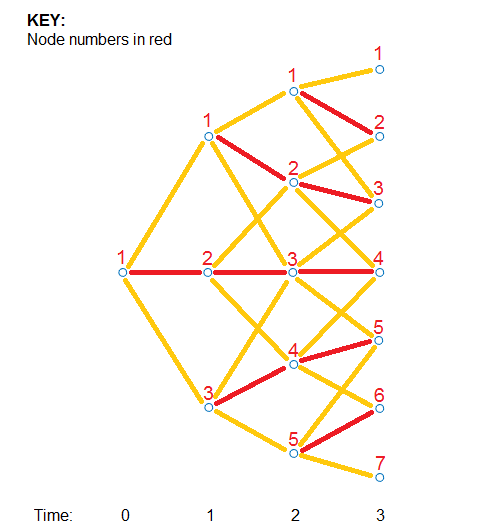The shape in the figure is the same shape obtained by running the function `treeviewer(HWT)`.

`Probs` Field

Using the following illustration, consider that you want to know the probability at t = `1` (second level of the tree) that the top node takes of one of the three paths.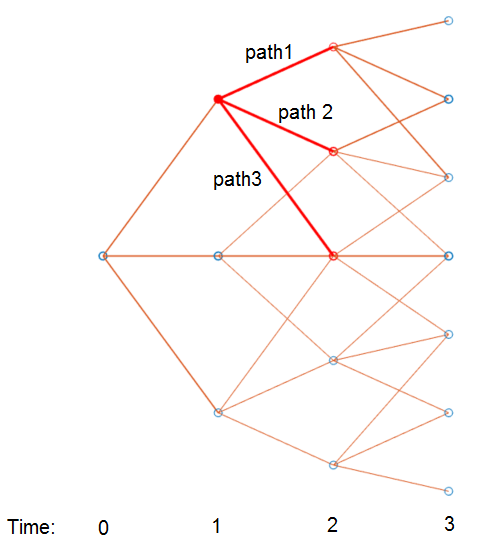`HWT.Probs` gives the probabilities that a particular branch has of moving from a given node to a node on the next level of the tree.

`HWT.Probs`
```ans=1×3 cell array {3x1 double} {3x3 double} {3x5 double} ```

The `Probs` field consists of a cell array with one cell per level of the tree. Find the probabilities of all three nodes at t = `1`, which corresponds to the second level of the tree.

`HWT.Probs{2}`
```ans = 3×3 0.1429 0.1667 0.1929 0.6642 0.6667 0.6642 0.1929 0.1667 0.1429 ```

Each column represents a different node. The first node at t = `1` corresponds to the first column and the probabilities are 14.29%, 66.42%, and 19.30%.

The probability of moving up (path 1) is the top value (14.29%), the middle path is the middle value (66.42%), and the path going down (path 3) is the bottom value in the array (19.30%). The following diagram summarizes this information.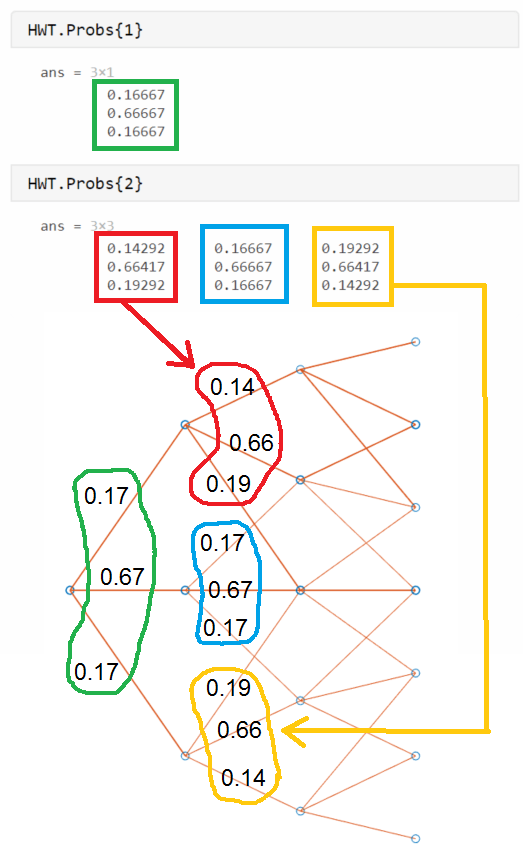### Examine the `PriceTree` Structure

The output of the pricing function is `PriceTree`. `PriceTree` has the following fields.

• `PriceTree.PTree` — contains the clean prices of each instrument.

• `PriceTree.ExTree` — contains the exercise indicator arrays where a value of `1` indicates the option has been exercised and a value of `0` indicates the option has not been exercised.

• `PriceTree.ExProbTree` — contains the exercise probabilities. A value of 0 indicates there was no exercise and a nonzero value gives the probability of reaching that node where the exercise happens.

• `PriceTree.ProbTree` — contains the probability tree indicating how likely any node is reached from the root node.

• `PriceTree.ExProbsByTreeLevel` — contains the exercise probability for a given option at each tree observation time. This is an aggregated view of `PriceTree.ExProbTree` that sums the values along all nodes at a particular time.

Note that for `ProbTree`, `PTree`, `ExTree`, and `ExProbTree`, each cell represents a different time in the tree, and inside each cell is an array. Each column in the array represents a different node on the tree at that particular tree level. This structure is the same as in `HWT.Probs`. However, for `PTree`, `ExTree`, and `ExProbTree` each row represents a different instrument. Because this example prices two instruments, there are only two rows. `ProbTree` contains only one row as the probability of reaching a particular node is independent of the instrument being priced.

Looking at `PriceTree.ProbTree`, examine the probabilities of reaching each of the five nodes from the root node at t = `2`, which is the third level of the tree.

`PriceTree.ProbTree{3}`
```ans = 1×5 0.0238 0.2218 0.5087 0.2218 0.0238 ```

These results are displayed in the following diagram, where all nodes are overlayed with their probabilities. The root node at t = `0` always has a probability of being reached, hence, it has a value of `1`.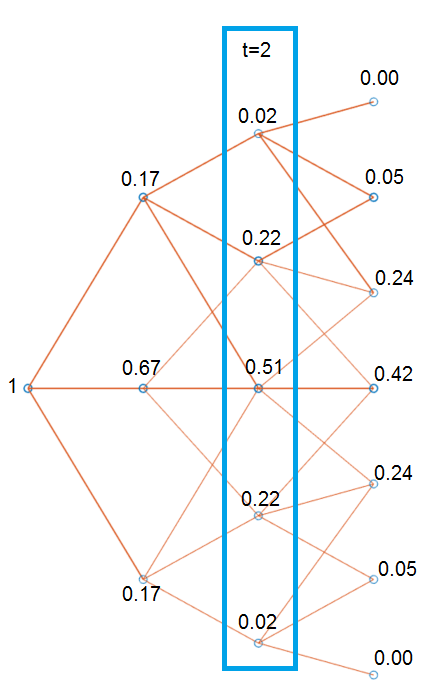By looking at `PriceTree.ExTree`, you can determine if the options are exercised. If either of the two instruments has options exercised at t = `2`, which is the third level of the tree, the values in `ExTree` are `1`; otherwise, the value is `0`.

`PriceTree.ExTree{3}`
```ans = 2×5 0 0 1 1 1 0 0 0 0 0 ```

At t = `2`, the first instrument has its option exercised at some nodes, while there is no exercise for the second instrument. The following diagram summarizes the exercise indicators on the tree.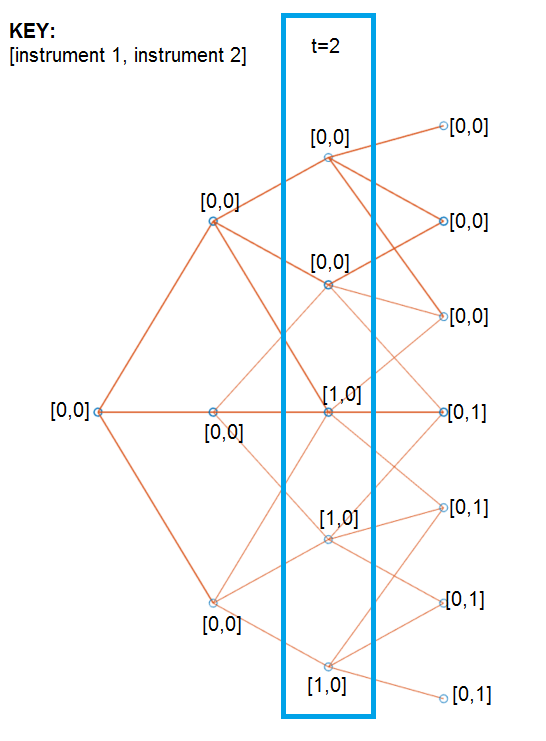Examine `ExProbTree`, which contains the exercise probabilities. These values indicate the probability of exercising the option.

`PriceTree.ExProbTree{3}`
```ans = 2×5 0 0 0.5087 0.2218 0.0238 0 0 0 0 0 ```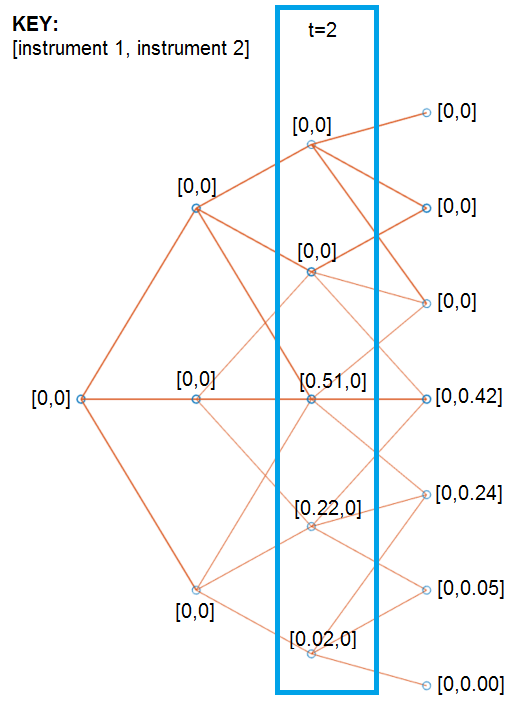`ExProbsByTreeLevel` is an aggregated view of `ExProbTrees`. Examine the exercise probabilities for the two options at each tree observation time.

`PriceTree.ExProbsByTreeLevel`
```ans = 2×5 0 0 0.7544 0 0 0 0 0 0.7124 0 ```

The first row corresponds to instrument 1, and the second row corresponds to instrument 2.

You can use `treeviewer` to examine the `tree` and `PriceTree` structures for any of the following tree types: